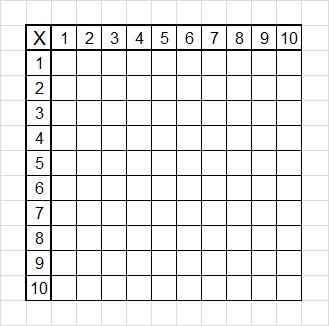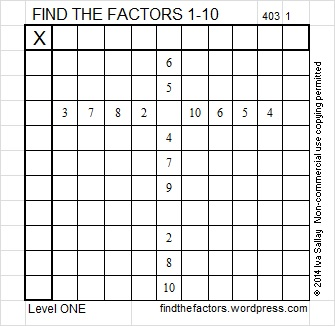# 31 and Every Third Grader Should Know

• 31 is a prime number.
• Prime factorization: 31 is prime.
• The exponent of prime number 31 is 1. Adding 1 to that exponent we get (1 + 1) = 2. Therefore 31 has exactly 2 factors.
• Factors of 31: 1, 31
• Factor pairs: 31 = 1 x 31
• 31 has no square factors that allow its square root to be simplified. √31 ≈ 5.56776How do we know that 31 is a prime number? If 31 were not a prime number, then it would be divisible by at least one prime number less than or equal to √31 ≈ 5.6. Since 31 cannot be divided evenly by 2, 3, or 5, we know that 31 is a prime number.

31 is never a clue in the FIND THE FACTORS puzzles.

Every third grader should know how to fill in a multiplication table:By the middle of third grade, every third grade student has been taught the basic multiplication facts. Of course, that doesn’t mean that every third grader has LEARNED those facts. Corestandards.org/Math/Content/3/ lists EVERYTHING a student should learn in third grade math. Multiplication is very key in learning all of these concepts.

Many students, even beyond third grade, rely on skip counting to figure out what a multiplication product should be. Some students have learned rhymes to help them recall a fact. Given the opportunity some students even in middle school will use a calculator to find the answer to a basic multiplication fact.

Practicing every day will help students recall the facts instantly instead of needing a calculator or other crutch to help them remember one of the basic multiplication facts. For variety, every third grader should also know how to fill out a multiplication table that looks like this:This kind of table doesn’t allow a student to simply count by twos, fives, nines or any other number to fill out a particular row or column. This type of table encourages a student to fully memorize all the multiplication facts so the table can be completed as quickly as possible.

About the middle of third grade, students are introduced to division. As soon as students learn that any number can be divided by 1, they are ready to tackle the easiest of the easiest FIND THE FACTORS puzzles. Here is the level 1 puzzle for the 3rd full week of 2014:It is a level 1 puzzle because students only have to know 10 (or 12) division facts to solve the puzzle. Even though it is a simple puzzle, third grade students will need some instruction to complete it. Tell them that this puzzle is going to turn into a multiplication table as soon as they figure out what numbers go into the top row and the first column. Tell them the only numbers that will be written in those two places are numbers from 1 to 10 and that all of those numbers have to be written in both places.

Help the students know what numbers to write in each space on the top row. Some students will likely be confused when they get to the column with 8 clues and to the column with no clues. Help them use logic to figure out what numbers should go in those spaces. When all the factors have been found in both the factor row and the factor column, have them complete the multiplication table. It will be great practice for all of them.

Last week’s puzzle was fairly easy. Here is the puzzle with all of the factors found:

This week’s puzzles are also available in an excel file here, if you have a spreadsheet program on your computer you can access it.

If you enable editing in excel, you can type your answers directly onto the puzzle, and you can also easily print the puzzles. Have fun!

## 3 thoughts on “31 and Every Third Grader Should Know”

1.wolfmarionette

These puzzles are really neat. I might give these to my sisters, so they can have some extra practice. 🙂

•ivasallay

Thank you! If your sisters are in elementary school, the level 1 or level 2 puzzles will be good practice. If they want more of a challenge, you can introduce level 3 or higher. I hope they like doing them!

•wolfmarionette

Okay, thanks! 🙂

This site uses Akismet to reduce spam. Learn how your comment data is processed.